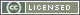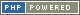# Interpreting types as abstract values

We expound a view of type checking as evaluation with `abstract values'. Whereas dynamic semantics, evaluation, deals with (dynamic) values like 0, 1, etc., static semantics, type checking, deals with approximations like `int`. A type system is sound if it correctly approximates the dynamic behavior and predicts its outcome: if the static semantics predicts that a term has the type `int`, the dynamic evaluation of the term, if it terminates, will yield an integer.

As object language, we will use simply typed and let-polymorphic lambda calculi with constants (integers and integer operations). We will use Haskell as a metalanguage: the language to write evaluators, type checkers, type reconstructors for the object language.

Just as our evaluator avoids the explicit environment in favor of substitutions, our type checker avoids the explicit type environment. This technique is particularly suitable if the object language is represented in higher-order abstract syntax (HOAS).

## Very tentative syllabus

• Define lambda calculus with constants in Haskell. Discuss different choices of representing variables: as strings, as de Bruijn indices, as HOAS. Environment-based vs. substitution-based evaluator.
• Introduce the simple type system; require binders to be annotated with types. Introduce types as abstract values, and type checking as evaluation. Discuss soundness of the type system: the fact the type checking relation is literally the extension of the evaluation relation makes soundness (or unsoundness) easier to see and grasp.
• Add Let to the calculus, discuss type schemas, drop the requirement all binders be annotated with types, and extend the type checking algorithm to do the inference. We now have to `guess' the annotations on the binders that were present before. For that, we develop a lightweight logic system, introduce substitutions and unification. Discuss how we manage to do inference without explicit type environments and how we can avoid explicit generalization. When type checking the let-form, show the difference between typecheck-then-substitute vs substitute-then-typecheck and discuss polymorphism as inlining.
• Define the representation of lambda terms that are typed by definition. Let the type system of Haskell itself do the type checking and the inference for us. Two approaches: initial (GADT) and final.
• Revisit type checking: converting from an untyped representation of lambda terms to the typed one: `typing dynamic typing'. There are lots of parallels with logic: weakening becomes explicit.

# Course Materials